Pathways through the Windows 7 MBR
(with Notes for Using the "Bochs Enhanced Debugger")

If you need any help in setting up the Bochs Debugger, please email us.

•  Major Sections of the MBR Code are:
•  Footnotes

This page describes each of the pathways that executing the Windows 7 MBR could possibly take, and what conditions might 'trigger' the code to go down one path or another. We will note where those decisions are made, so until you read otherwise, every instruction below will always be executed once the BIOS has loaded the MBR into Memory and transferred control of the PC's processor to it.

1. Copy the Code to Memory Locations 0x600 through 0x7FF

As we discussed on our Bochs Debugger page, Bochs will always "break" at the first instruction of its own BIOS code whenever it's run; which is also true when using the 'Enhanced Debugger,' so the first thing we need to do is ENTER: lb 0x7c00 and then a c in its command line (just above the word "Break" at the lower-left corner of the window). Once you ENTER the 'c' (to Continue), the Bochs BIOS will load the MBR code into Memory at 0x7c00 and wait for you to give it another command or press a key/button: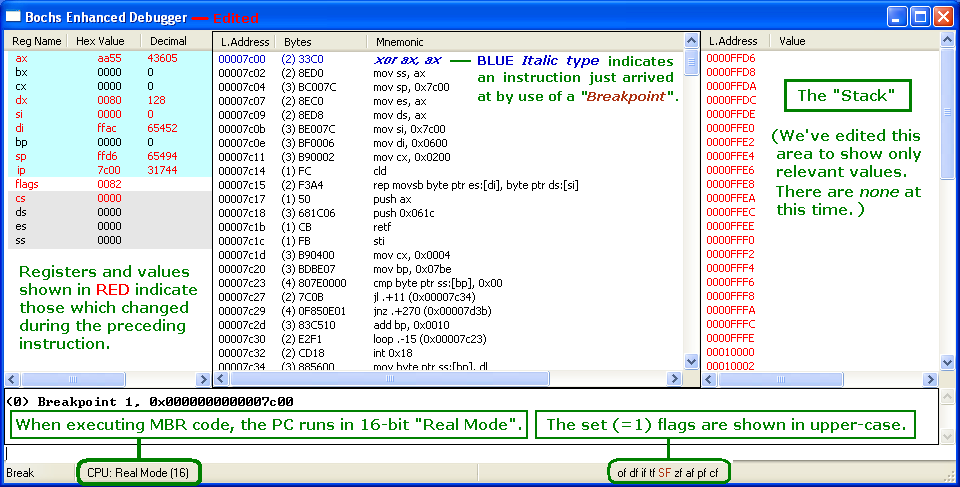Bochs normally displays 32-bit registers, but we've edited the screen to show only 16-bit registers (like MS-DEBUG).
Your screen will also display an eflags register like this:
id vip vif ac vm rf nt IOPL=0 of df if tf SF zf af pf cf

There's a great deal of information here! We're ready to execute the first MBR instruction in Memory at 0x7c00; code shown in BLUE italics indicates we have just arrived at a 'Breakpoint,' which will turn brown after being executed (all enabled Breakpoints are indicated by BROWN italic type). Among other things, the BIOS put AA55 in the AX register, 80h in the DL register, and left the Stack Pointer (SP) set to FFD6. Note: The CPU mode is always set to 16-bit REAL for execution of the MBR code. Only the SF (Sign) flag was set to 1; making it 'Negative' (flags register = 0082h). See our page on the 8086 CPU Registers (including how to interpret the "FLAGS" Register).

After entering an s on the command line to step into (execute) the first instruction, the one at 0x7c02 turns bold GREEN; indicating it will be the next instruction to be executed. Of course, the AX register was cleared to zero (by: xor ax,ax), the Sign flag was reset to 0 and both the ZF (Zero) and PF (Parity) flags have been set to 1 (flags register = 0046h):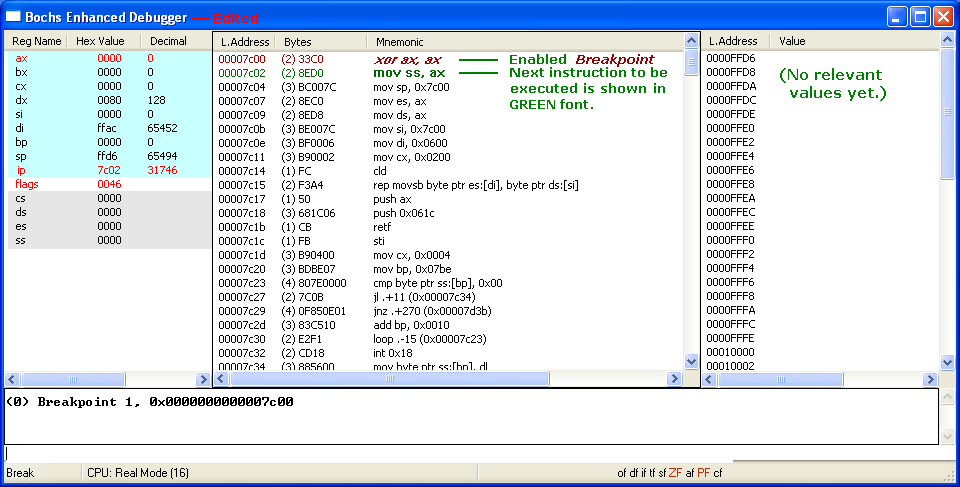mov ss, ax copies the zeros from AX into the Stack Segment (SS) Register; but it already was zero, so no real change there: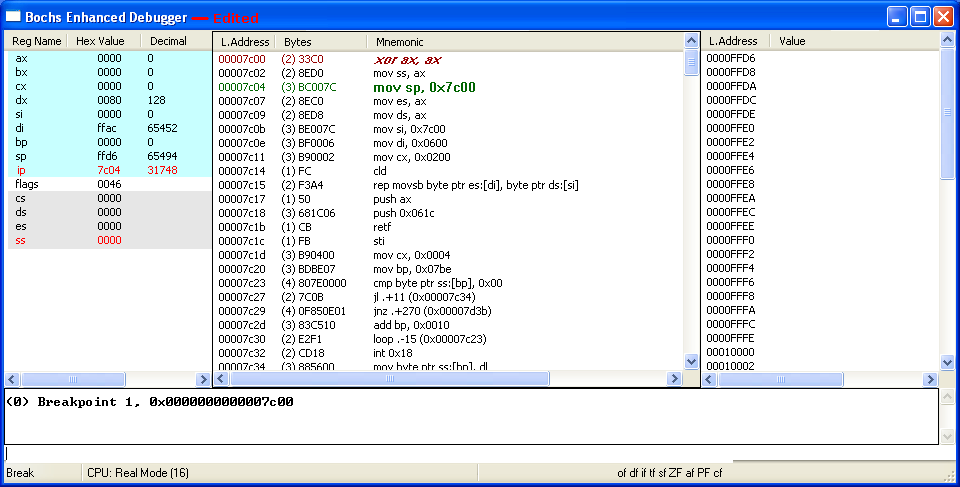mov sp, 0x7c00 changes the Stack Pointer (SP) to 0x7c00, and you see all the bytes of the MBR sector we're executing show up there (in RED):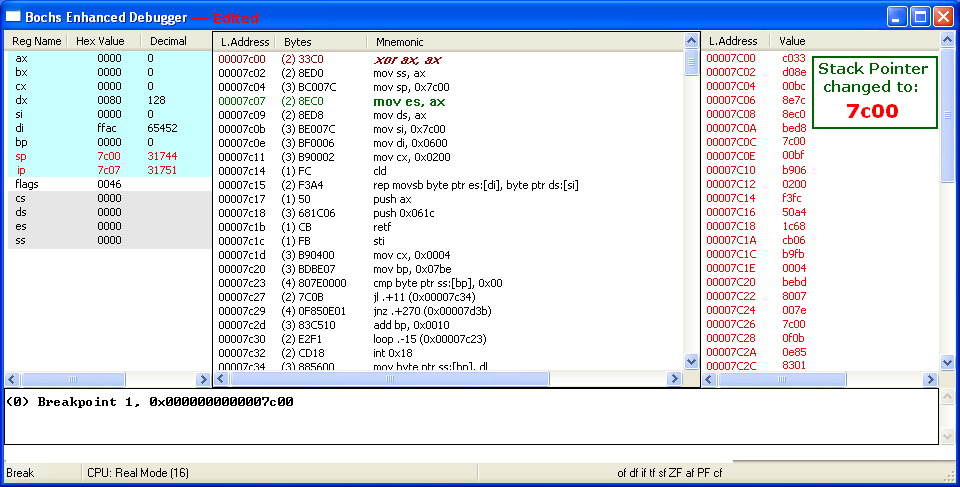NOTE: From this point on, for instructions in which very little changes, we'll provide only the following 'simplified text display' which is similar to that of the old MS-DOS DEBUG screen (but it shows both the Bochs and DEGUB Flags names).

 ```mov es,ax copies the zeros from AX into the ES (Extra Segment) Register; which was already zero, so no real change in ES: AX=0000 BX=0000 CX=0000 DX=0080 SP=7C00 BP=0000 SI=0000 DI=FFAC DS=1B17 ES=0000 SS=0000 CS=0000 IP=7C09 of df if sf ZF af PF cf 7C09 8ED8 MOV DS,AX NV UP DI PL ZR NA PE NC mov ds,ax copies the zeros from AX into the DS (Data Segment) Register; which was already zero, so again no actual change: AX=0000 BX=0000 CX=0000 DX=0080 SP=7C00 BP=0000 SI=0000 DI=FFAC DS=0000 ES=0000 SS=0000 CS=0000 IP=7C0B of df if sf ZF af PF cf 7C0B BE007C MOV SI,7C00 NV UP DI PL ZR NA PE NC mov si,7c00 puts 7C00 into the Source Index (SI) Register, because we want to copy all the code from 7c00 thru 7dff to a new location: AX=0000 BX=0000 CX=0000 DX=0080 SP=7C00 BP=0000 SI=7C00 DI=FFAC DS=0000 ES=0000 SS=0000 CS=0000 IP=7C0E of df if sf ZF af PF cf 7C0E BF0006 MOV DI,0600 NV UP DI PL ZR NA PE NC mov di,0600 puts 0600 into the Destination Index (DI) Register, since that's the new location in Memory we want to copy the MBR code to: AX=0000 BX=0000 CX=0000 DX=0080 SP=7C00 BP=0000 SI=7C00 DI=0600 DS=0000 ES=0000 SS=0000 CS=0000 IP=7C11 of df if sf ZF af PF cf 7C11 B90002 MOV CX,0200 NV UP DI PL ZR NA PE NC mov cx,0200 puts 0200 (hex) into the Count Register (CX), in order to copy all 512 (decimal) bytes of the code to locations 600 - 6ff: AX=0000 BX=0000 CX=0200 DX=0080 SP=7C00 BP=0000 SI=7C00 DI=0600 DS=0000 ES=0000 SS=0000 CS=0000 IP=7C14 of df if sf ZF af PF cf 7C14 FC CLD NV UP DI PL ZR NA PE NC```

The Clear Direction (cld) instruction ensures the Direction Flag (df) is set to zero, so that any string operations will INCREMENT the SI and/or DI Registers; that is, count up rather than down (decrement), since we want to copy the code to increasing memory locations (not decreasing ones). But df was already zero (indicating up), so it doesn't change: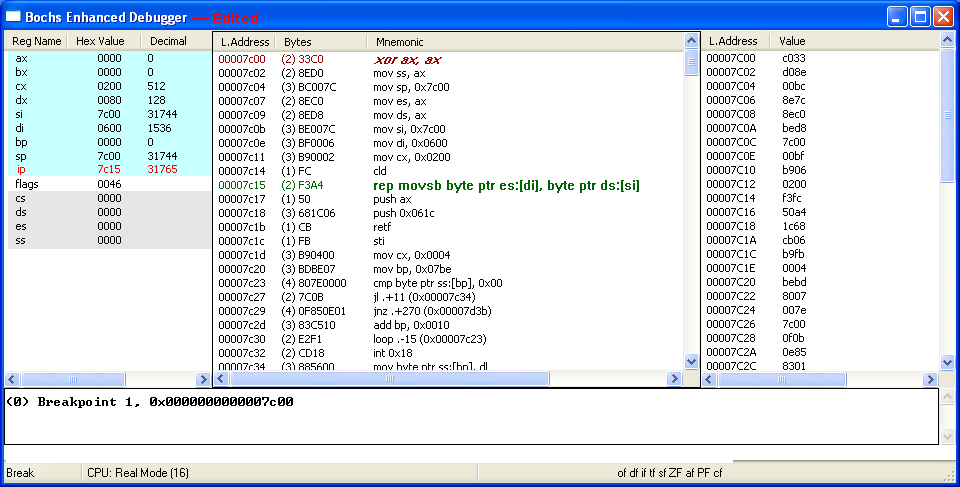The Bochs Debugger disassembles the machine code bytes F3 A4 as: rep movsb byte ptr es:[di], byte ptr ds:[si], which helps us humans to understand this will REPeatedly copy a string of bytes, one byte at a time, from the location pointed to by the DS:SI (Data Segment:Source Index) registers to the location pointed to by the ES:DI (Extra Segment:Destination Index) registers. And from the previous instructions, we know it will do so for 512 times (CX=0200), in an upwards direction. (See our notes on the REP Instruction in Microsoft's MBR code for some side details.)

The following shows how executing this rep movsb instruction in a step-by-step manner would take 512 steps! We're going to step through only the first four and finish with the last four (showing only 8 of the 512 steps). The CX register decrements, and the SI and DI registers will increment. The previous step copied the byte 33h from 7c00 to 0600 and each successive step will copy another of the MBR; until all 512 bytes are copied:

 ```AX=0000 BX=0000 CX=01FF DX=0080 SP=7C00 BP=0000 SI=7C01 DI=0601 DS=0000 ES=0000 SS=0000 CS=0000 IP=7C15 of df if sf ZF af PF cf 7C15 F3 REP NV UP DI PL ZR NA PE NC 7C16 A4 MOVSB AX=0000 BX=0000 CX=01FE DX=0080 SP=7C00 BP=0000 SI=7C02 DI=0602 DS=0000 ES=0000 SS=0000 CS=0000 IP=7C15 of df if sf ZF af PF cf 7C15 F3 REP NV UP DI PL ZR NA PE NC 7C16 A4 MOVSB AX=0000 BX=0000 CX=01FD DX=0080 SP=7C00 BP=0000 SI=7C03 DI=0603 DS=0000 ES=0000 SS=0000 CS=0000 IP=7C15 of df if sf ZF af PF cf 7C15 F3 REP NV UP DI PL ZR NA PE NC 7C16 A4 MOVSB AX=0000 BX=0000 CX=01FC DX=0080 SP=7C00 BP=0000 SI=7C04 DI=0604 DS=0000 ES=0000 SS=0000 CS=0000 IP=7C15 of df if sf ZF af PF cf 7C15 F3 REP NV UP DI PL ZR NA PE NC 7C16 A4 MOVSB ============================================== At this point we switch to the last four steps: ============================================== AX=0000 BX=0000 CX=0004 DX=0080 SP=7C00 BP=0000 SI=7DFC DI=07FC DS=0000 ES=0000 SS=0000 CS=0000 IP=7C15 of df if sf ZF af PF cf 7C15 F3 REP NV UP DI PL ZR NA PE NC 7C16 A4 MOVSB AX=0000 BX=0000 CX=0003 DX=0080 SP=7C00 BP=0000 SI=7DFD DI=07FD DS=0000 ES=0000 SS=0000 CS=0000 IP=7C15 of df if sf ZF af PF cf 7C15 F3 REP NV UP DI PL ZR NA PE NC 7C16 A4 MOVSB AX=0000 BX=0000 CX=0002 DX=0080 SP=7C00 BP=0000 SI=7DFE DI=07FE DS=0000 ES=0000 SS=0000 CS=0000 IP=7C15 of df if sf ZF af PF cf 7C15 F3 REP NV UP DI PL ZR NA PE NC 7C16 A4 MOVSB AX=0000 BX=0000 CX=0001 DX=0080 SP=7C00 BP=0000 SI=7DFF DI=07FF DS=0000 ES=0000 SS=0000 CS=0000 IP=7C15 of df if sf ZF af PF cf 7C15 F3 REP NV UP DI PL ZR NA PE NC 7C16 A4 MOVSB```

NOTE: You can skip all 512 single steps above by simply entering: lb 0x7c17 and then c at the Command line; which will take you directly to the following instruction.

The following shows the results of executing the last step above (the CX register becomes zero, so we can move on to the next group of instructions which will push data onto the Stack):

 ```AX=0000 BX=0000 CX=0000 DX=0080 SP=7C00 BP=0000 SI=7E00 DI=0800 DS=0000 ES=0000 SS=0000 CS=0000 IP=7C17 of df if sf ZF af PF cf 7C17 50 PUSH AX NV UP DI PL ZR NA PE NC```

The next two screens show the zero bytes of the AX register and the Word 0x061c being pushed onto the Stack: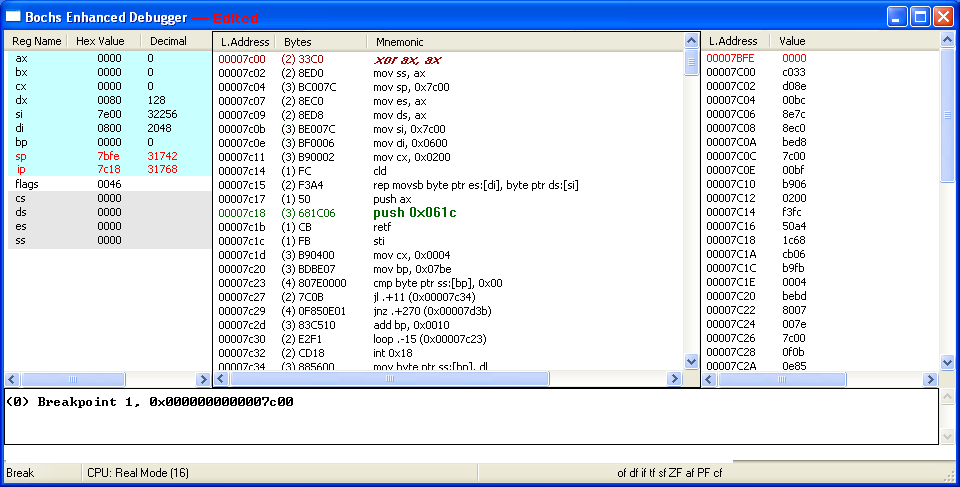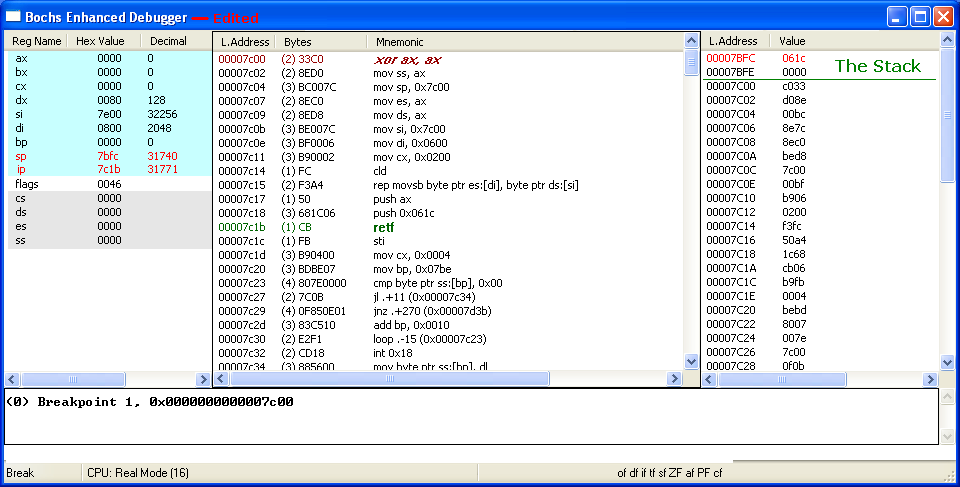And with the execution of the retf (Return Far) instruction, both the Words we pushed onto the Stack are removed from it, and we begin executing the next instruction at its new location of 0000:061C (the whole sector having been copied to 0x600 through 0x7FF).

2. Checking for an 'Active'1 Partition

After starting your Bochs Debugger session on an image file with a Windows 7 MBR sector, you can of course, jump right to the following display, by entering: lb 0x61c and then c at the Enhanced Debugger's Command line:

 ```AX=0000 BX=0000 CX=0000 DX=0080 SP=7C00 BP=0000 SI=7E00 DI=0800 DS=0000 ES=0000 SS=0000 CS=0000 IP=061C of df if sf ZF af PF cf 061C FB STI NV UP DI PL ZR NA PE NC```

The sti (Set Interrupt Flag) sets this bit (IF = 1) in the Flags Register, enabling interrupts; MS-DEBUG's flag indicator will change from "DI" (Disable Interrupts) to "EI" (Enable Interrupts). This means the processor begins responding to external, maskable interrupts after the next instruction is executed. Apart from other changes noted in the following instructions, the hex Word for the Flags Register becomes: 0246.

 ```AX=0000 BX=0000 CX=0000 DX=0080 SP=7C00 BP=0000 SI=7E00 DI=0800 DS=0000 ES=0000 SS=0000 CS=0000 IP=061C of df IF sf ZF af PF cf 061D B90400 MOV CX,0004 NV UP EI PL ZR NA PE NC mov cx,0004 changes the Count (CX) Register; so if needed, all four Partition Table entries will be checked for the Active indicator (80h): AX=0000 BX=0000 CX=0004 DX=0080 SP=7C00 BP=0000 SI=7E00 DI=0800 DS=0000 ES=0000 SS=0000 CS=0000 IP=0620 of df IF sf ZF af PF cf 0620 BDBE07 MOV BP,07BE NV UP EI PL ZR NA PE NC mov bp,07be puts 07be into the Base Pointer (BP) Register, so we can check for 80h at the correct offset in each of the 4 P. Table entries: AX=0000 BX=0000 CX=0004 DX=0080 SP=7C00 BP=07BE SI=7E00 DI=0800 DS=0000 ES=0000 SS=0000 CS=0000 IP=0623 of df IF sf ZF af PF cf NV UP EI PL ZR NA PE NC 0623 807E0000 CMP BYTE PTR [BP+00],00 SS:07BE=80```

This multi-byte CMP (CoMPare) instruction compares the value at the Memory location pointed to by the SS:BP registers and the given value (Zero), by: Subtracting the second operand (the Zero) from the first operand (that value in Memory), then setting or clearing bits in the Flags Register according to the rules for Subtraction (see How Flags are Set for more details). The only difference between this CMP instruction and its corresponding SUB instruction ("80 6E 00 00") is that the Subtraction operation will also store the result in the first operand.

The first byte in each of the four possible 16-byte Partition Table entries indicates whether that partition is the Active partition or not. If, as in the case of most Windows™ OS installs, the Active partition is the first one, then Memory location 0x7be will contain an 80 hex byte (as shown above); which results in the following: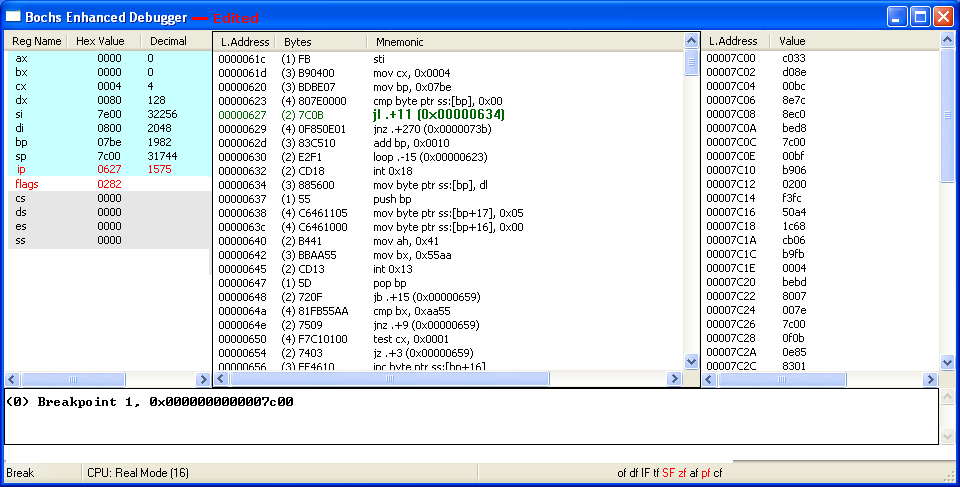Since 80h (at 0x7be) - 0 = 80h, the highest bit (bit 7) is a 1, so the Sign Flag is set (SF=1). The Zero Flag is reset (zf=0) since the result is not all zero bits, and the Parity Flag is also reset (pf=0) since there's only one 1's bit (an odd number) in the result; all other flags are unaffected. (Note: Any byte with its highest bit set indicates a Negative number and 2's Complement arithmetic; as explained on our 2-Byte Jumps page, must be used).

Take the following links to see what happens if:

A. The MBR finds a 01 through 7Fh byte

B. The MBR finds only zero bytes (No Active Entry)

C. The Last Partition is Active (No entry problems)

D. The MBR finds an 81h through FFh byte

E. Continue with First Entry as Active (80h in 0x7be):

The CMP instruction (just like Subtraction) uses signed bytes (80h actually represents a negative 128), thus the reason the comparison results in a LESS THAN condition; or expressed differently: Whenever SF (Sign Flag) is not equal to OF (Overflow Flag). So, execution jumps (forward; "+") by 11 bytes, to location 0x634:

 ```AX=0000 BX=0000 CX=0004 DX=0080 SP=7C00 BP=07BE SI=7E00 DI=0800 DS=0000 ES=0000 SS=0000 CS=0000 IP=0634 of df IF SF zf af pf cf NV UP EI NG NZ NA PO NC 0634 885600 MOV [BP+00],DL SS:07BE=80```

More will be posted in the near future... this page is still Under Construction !!!

If you need any help in setting up the Bochs Debugger, please email us.

Footnotes

1[Return to Text] The official Windows 7 OS install DVD creates 2 partitions on an un-partitioned drive: The first is labeled "System Reserved" (with a capacity of 100 MiB); it is set to be the Active partition (Active meaning the partition which 'boots-up' first; there can only be one of these). The other (largest) partition, contains the Windows 7 OS and is set with the drive letter C: and is also listed as the Boot partition as can be seen in Disk Management: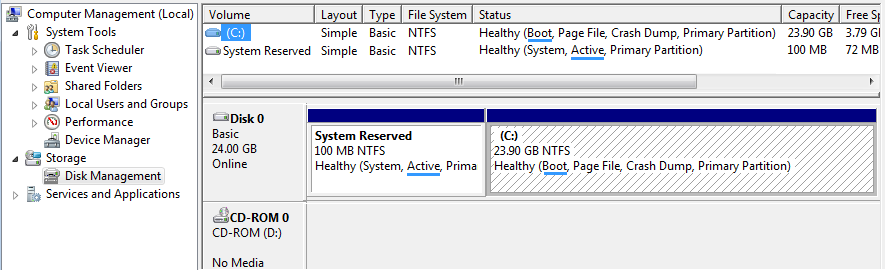Disk Management view of small 25 GB Win 7 OS drive. The terms Active and Boot no longer refer to the same partition.

The term Boot refers to the partition which contains the operating system which will boot-up; not the first "Boot Sector" that is loaded into Memory and executed, which in this case actually comes from the Active partition. [Return to Text]

Date Page Added: May 19, 2013 (19.05.2013).
Updated: May 20, 2013 (20.05.2013); May 26, 2013 (26.05.2013); May 27, 2013 (27.05.2013); June 28, 2013 (28.06.2013).
Last Updated: July 5, 2013 (05.07.2013).

You can write to us using this: online reply form. (It opens in a new window.)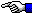The Starman's x86 ASSEMBLY PagesThe Starman's Realm Index Page